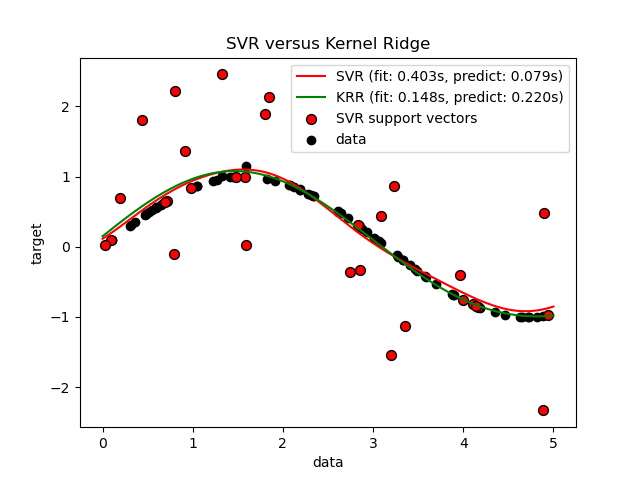# sklearn.model_selection.learning_curve¶

sklearn.model_selection.learning_curve(estimator, X, y, *, groups=None, train_sizes=array([0.1, 0.33, 0.55, 0.78, 1. ]), cv=None, scoring=None, exploit_incremental_learning=False, n_jobs=None, pre_dispatch='all', verbose=0, shuffle=False, random_state=None, error_score=nan, return_times=False)

[源码]

estimator object type that implements the “fit” and “predict” methods

X array-like of shape (n_samples, n_features)

y array-like of shape (n_samples,) or (n_samples, n_outputs)

groups array-like of shape (n_samples,), default=None

train_sizes array-like of shape (n_ticks,), default=np.linspace(0.1, 1.0, 5)

cv int, cross-validation generator or an iterable, default=None

- None，默认使用5折交叉验证
- int，用于指定(Stratified)KFold的折数
- CV splitter,
- 可迭代输出训练集和测试集的切分作为索引数组

scoring str or callable, default=None

exploit_incremental_learning bool, default=False

n_jobs int, default=None

pre_dispatch int or str, default=’all’

verbose int, default=0

shuffle bool, default=False

random_state int or RandomState instance, default=None
shuffle为true时使用。为多个函数调用传递可重复输出的int值。请参阅词汇表
error_score ‘raise’ or numeric, default=np.nan

0.20版中的新功能。
return_times bool, default=False

train_sizes_abs array of shape (n_unique_ticks,)

train_scores array of shape (n_ticks, n_cv_folds)

test_scores array of shape (n_ticks, n_cv_folds)

fit_times array of shape (n_ticks, n_cv_folds)

score_times array of shape (n_ticks, n_cv_folds)

## sklearn.model_selection.learning_curve使用示例¶